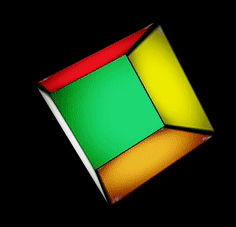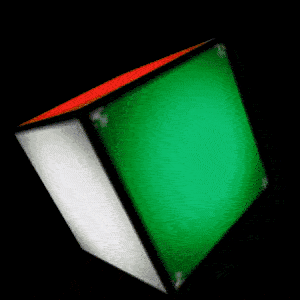# OpenGL 深度测试与精度值的那些事

##### 标签: OpenGLgl_FragCoord 的 x 和 y 分量代表了片段的屏幕空间坐标（其（0，0）位于左下角）。gl_FragCoord 中也包含了一个 z 分量，它包含了片段真正的深度值。z 值就是需要与深度缓冲内容所对比的那个汁。

glEnable(GL_DEPTH_TEST);


// 清除深度缓冲
glClear(GL_COLOR_BUFFER_BIT | GL_DEPTH_BUFFER_BIT);


OpenGL 运行我们禁用深度缓冲的写入，只需要设置它的深度掩码为 GL_FALSE 即可。

// 设置只读的深度缓冲


## 深度测试函数

OpenGL 允许修改深度测试中使用的比较运算符，允许我们控制 OpenGL 什么时候该通过或丢弃一个片段，什么时候更新深度缓冲。

glDepthFunc(GL_LESS);


GL_ALWAYS 永远通过深度测试
GL_NEVER 永远不通过深度测试
GL_LESS 在片段深度值小于缓冲的深度值时通过测试
GL_EQUAL 在片段深度值等于缓冲区的深度值时通过测试
GL_LEQUAL 在片段深度值小于等于缓冲区的深度值时通过测试
GL_GREATER 在片段深度值大于缓冲区的深度值时通过测试
GL_NOTEQUAL 在片段深度值不等于缓冲区的深度值时通过测试
GL_GEQUAL 在片段深度值大于等于缓冲区的深度值时通过测试

glEnable(GL_DEPTH_TEST);
glDepthFunc(GL_ALWAYS);## 深度值精度

$$\begin{equation} F_{depth} = \frac{z - near}{far - near} \end{equation}$$$$\begin{equation} F_{depth} = \frac{1/z - 1/near}{1/far - 1/near} \end{equation}$$https://github.com/glumes/AndroidOpenGLTutorial

## 参考

「真诚赞赏，手留余香」#### 欢迎扫码关注微信公众号：【音视频开发进阶】，获得最新文章推送~~~